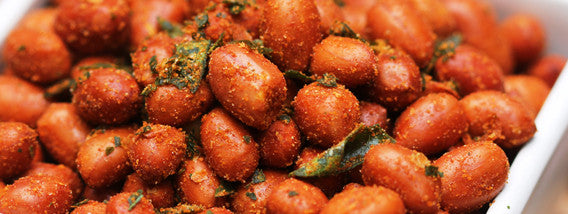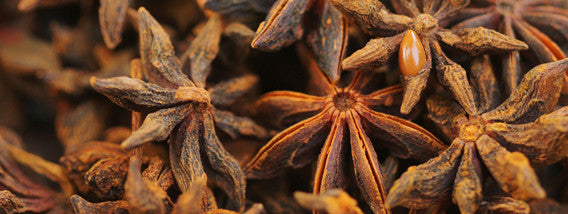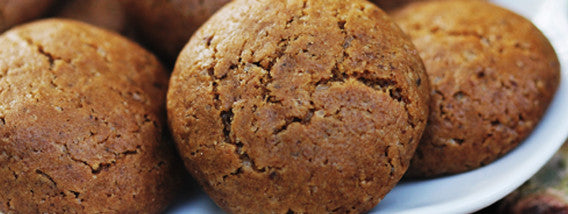Rs. 80
500g
Rs. 105
500g
Rs. 50
250g
Rs. 125
400g
Rs. 130
500g
Rs. 55
500g
Rs. 55
250g
Rs. 42
500g
Rs. 50
500g
Rs. 70
500g
Rs. 75
500g
Rs. 70
100g

Rs. 99
75g
Rs. 110
60g
Rs. 45
150g / 50g
Rs. 45
150g / 50g
Rs. 68
150g / 75g
Rs. 120
125g
Rs. 95
100g
Rs. 95
125g
Rs. 50
150g / 50g
Rs. 170
100g
Rs. 99
125g
Rs. 68
150g / 75g